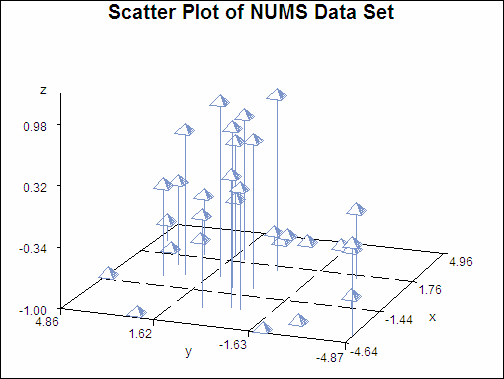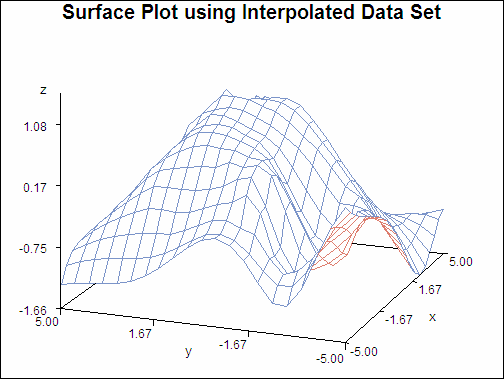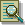The G3GRID Procedure

# Example 1: Using the Default Interpolation Method

Procedure features:

G3GRID statement options:

 OUT=

GRID statement options:

 AXIS1= AXIS2=
Other features:
 DATA step G3D procedure
Sample library member: GTGDEFIN

Scatter Plot of NUMS Data Set (gtgdefin)This example demonstrates the default interpolation method that is used by the GRID statement. The example first generates a scatter plot of random data to show the concentration of data values before processing the data set with the G3GRID procedure. The original data does not contain enough combinations of x, y and z values to:

• generate a surface plot with the G3D procedure

• generate a contour plot with the GCONTOUR procedure

The example then runs the G3GRID procedure to interpolate additional x, y, and z values. Because no interpolation method is specified, the default interpolation method is used. The resulting output data set is used as input to the G3D procedure, which generates the surface plot shown in the following output.

Surface Plot using Interpolated Data Set (gtgdefin)`goptions reset=all border;````data nums; keep x y z; do i=1 to 30; x=10*ranuni(33)-5; y=10*ranuni(35)-5; z=sin(sqrt(x*x+y*y)); output; end; run;````title "Scatter Plot of NUMS Data Set";````proc g3d data=nums; scatter y*x=z; run; quit;``````proc g3grid data=nums out=default; grid y*x=z / axis1=-5 to 5 by .5 axis2=-5 to 5 by .5; run; quit;````title "Surface Plot using Interpolated Data Set";````proc g3d data=default; plot y*x=z; run; quit;```Previous Page | Next Page | Top of Page